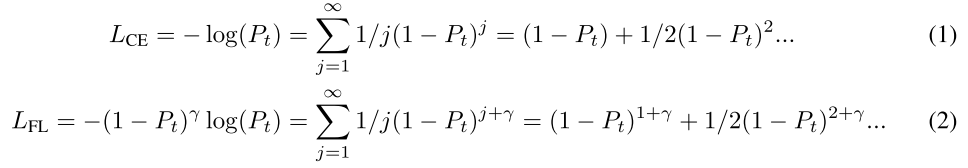# [Notes] PolyLoss: A Polynomial Expansion Perspective of Classification Loss Functions

## Introduction

Recall that an one-dimensional Taylor series is an expansion of a real function $f(x)$ about a point $x = a$ :

$$f(x) = f(a) + f'(a)(x-a) + \frac{f''(a)}{2!}(x-a)^2 + .. + \frac{f^{n}(a)}{n!}(x-a)^n + ...$$

We can approximate the cross-entropy loss using the Taylor series (a.k.a. Taylor expansion) using $a = 1$:

$$f(x) = -log(x) = 0 + (-1)(1)^{-1}(x-1) + (-1)^2(1)^{-2}\frac{(x-1)^2}{2} + ... \\ = \sum^{\infty}_{j=1}(-1)^j\frac{(j-1)!}{j!}(x-1)^{j} = \sum^{\infty}_{j=1}\frac{(1-x)^{j}}{j}$$

We can get the expansion for the focal loss simply by multiplying the cross-entropy loss series by $(1-x)^\gamma$:Log loss and Focal loss (Note: $x = P_t$)

The main idea of this paper is to adjust the polynomial coefficients for some of the polynomial bases $(1 - P_t)^j$, which are $1/j$ in the above expansions. Experiments show that adjusting the polynomial coefficient for the first polynomial base ($(1 - P_t)$ for cross-entropy loss and $(1 - P_t)^{\gamma}$ for focal loss) is enough to get a performance boosts while requires minimal hyper-parameter tuning (the paper calls it the Poly-1 formulation).

## How and why does it work

As you can see from the above equation, the gradient for the first polynomial term is a constant value of 1. That means the value of $P_t$ does not affect it at all. The sheer appearance of an example of that target class in the training batch will contribute a gradient of 1 from this term. In other words, this term is simply counting class occurrences and will be swayed by the majority class, especially when the dataset is highly imbalanced.

You’d think that we’d want to reduce the coefficient for the first term whenever the dataset is imbalanced. But it is not always the case. The paper shows that increasing the coefficient boost the accuracy on ImageNet-21K from 45.8 to 46.4. Their theory is that the model trained with cross-entropy loss is not confident enough, and putting more emphasis on the majority classes helps the model to gain confidence.

For another imbalanced dataset (COCO), reducing the coefficient of the first term to zero provides the best precision and recall. Therefore, there are generally no a rule of thumb for determining the value of the coefficient. You have to do hyper-parameter tuning to determine the best perturbation value $\epsilon$ for different datasets and tasks.

## PyTorch Implementations

Because only the first polynomial term is modified in Poly-1 losses, we can divide the loss into two parts: the regular loss value and the correcting value for the first term. Here’s an implementation of Poly-1 cross-entropy Loss published by Abdulla Huseynov:

class Poly1CrossEntropyLoss(nn.Module):
def __init__(self,
num_classes: int,
epsilon: float = 1.0,
reduction: str = "none",
weight: Tensor = None):
super(Poly1CrossEntropyLoss, self).__init__()
self.num_classes = num_classes
self.epsilon = epsilon
self.reduction = reduction
self.weight = weight
return

def forward(self, logits, labels):
labels_onehot = F.one_hot(labels, num_classes=self.num_classes).to(device=logits.device,
dtype=logits.dtype)
pt = torch.sum(labels_onehot * F.softmax(logits, dim=-1), dim=-1)
CE = F.cross_entropy(input=logits,
target=labels,
reduction='none',
weight=self.weight)
poly1 = CE + self.epsilon * (1 - pt)
if self.reduction == "mean":
poly1 = poly1.mean()
elif self.reduction == "sum":
poly1 = poly1.sum()
return


The core logic is in this line: poly1 = CE + self.epsilon * (1 - pt).

The above implementation use one-hot encoding to retrieve the values of $P_t$. I created a slightly different implementation in pytorch-lightning-spell using torch.gather:

class Poly1CrossEntropyLoss(nn.Module):
def __init__(
self,
epsilon: float = 1.0,
reduction: str = "none",
weight: Optional[Tensor] = None
):
super(Poly1CrossEntropyLoss, self).__init__()
self.epsilon = epsilon
self.reduction = reduction
self.weight = weight

def forward(self, logits, labels, **kwargs):
probs = F.softmax(logits, dim=-1)
if self.weight is not None:
self.weight = self.weight.to(labels.device)
probs = probs * self.weight.unsqueeze(0) / self.weight.mean()
pt = torch.gather(probs, -1, labels.unsqueeze(1))[:, 0]
CE = F.cross_entropy(
input=logits, target=labels,
reduction="none", weight=self.weight
)
poly1 = CE + self.epsilon * (1 - pt)
if self.reduction == "mean":
poly1 = poly1.mean()
elif self.reduction == "sum":
poly1 = poly1.sum()
return poly1


I also applied the optional class weights to the correcting value in this line: probs = probs * self.weight.unsqueeze(0) / self.weight.mean(). Without this line, the first coefficient will not be modified to zero when $\epsilon=-1$ with an uneven distribution of class weights.

The Poly-1 focal loss works similarly. You just need to add the $(1-pt)^{\gamma}$ term into the calculation. Please refer to Abdulla Huseynov’s implementation for more details.

1. Leng, Z., Tan, M., Liu, C., Cubuk, E. D., Shi, X., Cheng, S., & Anguelov, D. (2022). PolyLoss: A Polynomial Expansion Perspective of Classification Loss Functions.
2. Taylor Series on Wolfram MathWorld.
3. PolyLoss in Pytorch (abhuse/polyloss-pytorch).
4. PyTorch Lightning Spells (veritable-tech/pytorch-lightning-spells).
tweet Share